Energy Filtered Electron Diffraction/Electron Spectroscopic Diffraction
(Aangular Distribution of Inelastically Scattered Electrons)
- Practical Electron Microscopy and Database -
- An Online Book -

https://www.globalsino.com/EM/

=================================================================================

Electron spectroscopic diffraction (ESD) in an energy-filtering electron transmission microscope (EFTEM) is used to record electron diffraction patterns with selected energy windows.

Based on the theory described by Reimer etc. , the angular intensity distribution of inelastically scattered electrons from element i can be given by a two-dimensional convolution,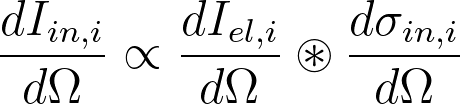------------------------- [3505a]
where,
dIel,i/dΩ -- angular intensity distribution of elastically scattered electrons of element i (see page4774),
dΩ = 2πθdθ (between θ and θ + dθ independent of azimuth),
dσin,i/dΩ -- angular distribution of inelastically scattered electrons of element i which can be approximated by,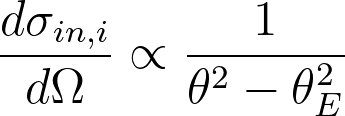(for θ < θc) ------------------------- [3505b]
= 0   (for θ > θc) ------------------------- [3505c]
where,
θE (≈ΔE/2E) --  the characteristic angle, which increases with increasing energy loss ΔE.
θc  -- (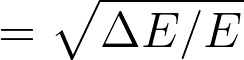) Compton angle, which is the cut-off angle of inelastic scattering and increases in diameter with increasing ΔE [2,3] .

For a complex material, the total elastic cross-section of the components in the material can be evaluated by summing all the cross-sections of the elements (see page4424).

Figure 3505a shows the radial intensity of the diffraction pattern of a 27 nm amorphous Ge film: (a) unfiltered, (b) zero-loss-filtered and (c) plasmon-loss-filtered (ΔE = 17 eV).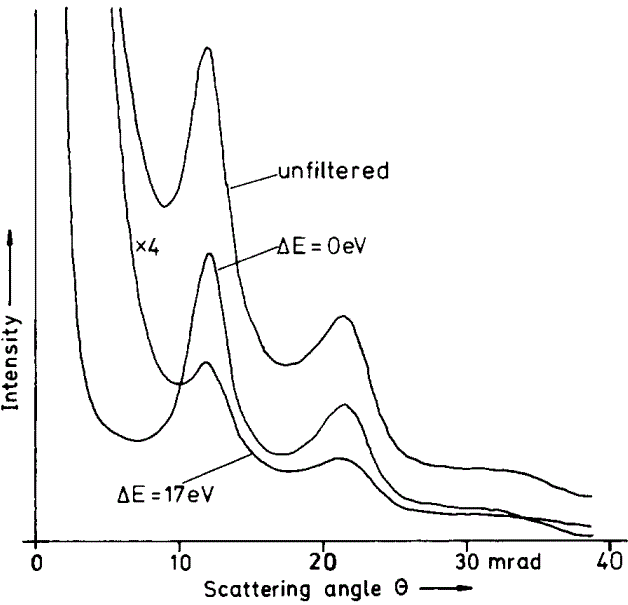Figure 3505a. Radial intensity of the diffraction pattern of a 27 nm amorphous Ge film: (a) unfiltered, (b) zero-loss-filtered and (c) plasmon-loss-filtered (ΔE = 17 eV). 

Figure 3505b shows two-dimensional (2D) convolution of a radial zero-loss intensity distribution by the inelastic differential cross section for energy losses of ΔE = 1 and 17 eV.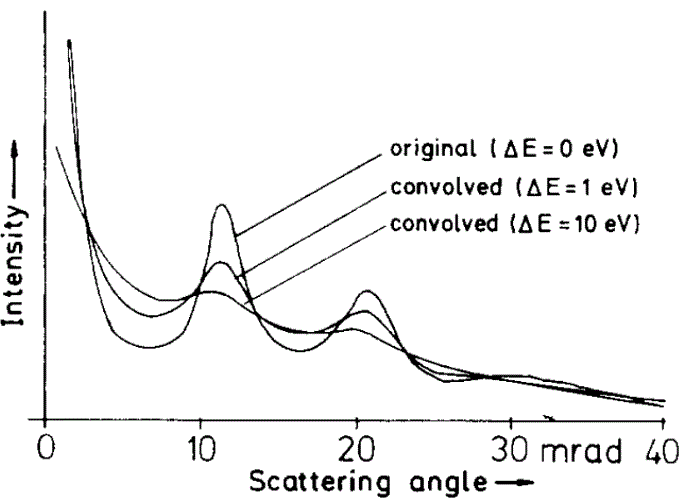Figure 3505b. 2D convolution of a radial zero-loss intensity distribution, of a 27 nm amorphous Ge film, by the inelastic differential cross section for energy losses of ΔE = 1 and 17 eV. 

 L. Reimer, I. Fromm, I. Naundorf, Electron spectroscopic diffraction, Ultramicroscopy, 32 (1990) 80-91.
 L. Reimer, 1. Fromm and R. Rennekamp, Ultramicroscopy 24 (1988) 339.
 L. Reimer and R. Rennekamp, Uhramicroscopy 28 (1989) 258.

=================================================================================